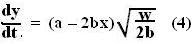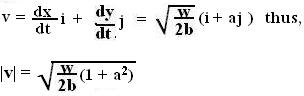## Tuesday, July 17, 2007

### Irodov Problem 1.27

First we start from the equation of the given curve and derive the relations between the time derivatives as below,We know however, that the body moves with constant acceleration of w along the -ive y-axis, this means that,Now we substitute (2) in (1b) to obtain,What (3) means is that, since there is no acceleration along the x-axis, the component of velocity of the point along the x-axis will remain constant at the value given by (3).

Now we can determine the value of dy/dt from (1a) as,The velocity of the particle at the origin is thus given by setting x=0, y=0 as,Anonymous said...

i have got another solution without using calculus
please tell me whether my aproach is correct or not
y=ax-bx^2
let the horizontal component of its velocity at the origin be Vx and vertical component of velocity at the origin be Vy
as the acceleration is along the negetive y direction
motion along the x direction is uniform. acceleration=-ω
eqn on motion along x direction
x=Vxt
along y direction
y=Vyt-1/2ωt^2
we know that
y=ax-bx^2
substituting for x we get y=a(Vxt)-b(Vxt)^2
equating we get
Vx=aVy
bVx^2=1/2ω
using these eqns to fing root of(Vx^2 + Vy^2)
which is the velocity at origin
= root of [(1+a^2)ω/2b ]

Krishna Kant Chintalapudi said...

This is also a valid way of doing it :-)